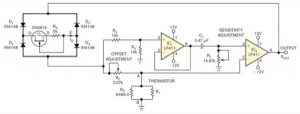# Temperature-to-period circuit provides linearization of thermistor response

Designers often use thermistors rather than other temperature sensors because thermistors offer high sensitivity, compactness, low cost, and small time constants. But most thermistors’ resistance-versus-temperature characteristics are highly nonlinear and need correction for applications that require a linear response. Using a thermistor as a sensor, the simple circuit in Figure 1 provides a time period varying linearly with temperature with a nonlinearity error of less than 0.1K over a range as high as 30K. You can use a frequency counter to convert the period into a digital output. An approximation derived from Bosson’s Law for thermistor resistance, RT, as a function of temperature, θ, comprises RT=AB–θ (see sidebar “Exploring Bosson’s Law and its equation”). This relationship closely represents an actual thermistor’s behavior over a narrow temperature range.You can connect a parallel resistance, RP, of appropriate value across the thermistor and obtain an effective resistance that tracks fairly close to AB–θ30K. In Figure 1, the network connected between terminals A and B provides an effective resistance of RABAB–θ. JFET Q1 and resistance RS form a current regulator that supplies a constant current sink, IS, between terminals D and E.

Through buffer-amplifier IC1, the voltage across R4 excites the RC circuit comprising R1 and C1 in series, producing an exponentially decaying voltage across R1 when R2 is greater than RAB. At the instant when the decaying voltage across R1 falls below the voltage across thermistor RT, the output of comparator IC2 changes its state. The circuit oscillates, producing the voltage waveforms in Figure 2 at IC2‘s output. The period of oscillation, T, is T=2R1C1ln(R2/RAB)2R1C1[ln(R2/A)+θlnB]. This equation indicates that T varies linearly with thermistor temperature θ.

READ  Voltage indicator transitions between colours

You can easily vary the conversion sensitivity, ΔT/Δθ, by varying resistor R1‘s value. The current source comprising Q1 and R1 renders the output period, T, largely insensitive to variations in supply voltage and output load. You can vary the period, T, without affecting conversion sensitivity by varying R2. For a given temperature range, θL to θH, and conversion sensitivity, SC, you can design the circuit as follows: Let θC represent the center temperature of the range. Measure the thermistor’s resistance at temperatures θL, θC, and θH. Using the three resistance values RL, RC, and RH, determine RP, for which RAB at θC represents the geometric mean of RAB at θL and θH. For this value of RP, you get RAB exactly equal to AB–θ at the three temperatures, θL, θC, and θH.